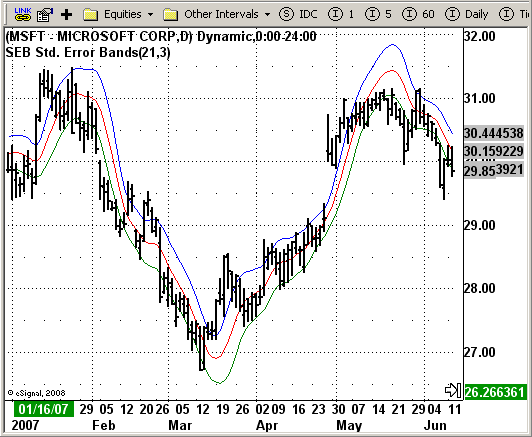# SEB - Standard Error Bands

ICE Data Services -

 stderrbands_orig.efs EFSLibrary - Discussion Board

File Name: stderrbands_orig.efs

Description:
This Indicator plots SEB Std Error bands indicator

Formula Parameters:
Length for regression line: 21
Length for standard errors: 3

Notes:
The construction of standard error bands is similar to that of Bollinger
bands. The difference: instead of measuring the variance or degree of volatility
around the average price using the standard deviation, standard error bands require
the use of the standard error of the estimate around a 21-period linear regression line.
The middle band is calculated as the ending value of a 21-period linear regression line
and adding two standard errors to the ending value of the regression line to form the
upper standard error band. To form the lower standard error band, subtract two standard
errors from the end value of the linear regression line.

This indicator is calculated according to this formula:

Upper Band = SmoothedRegressionLine (EndPointOfRegression of Close Price,SDeg) + SmoothedStdError
Lower Band = SmoothedRegressionLine (EndPointOfRegression of Close Price,SDeg) - SmoothedStdError`/*********************************Provided By: eSignal (Copyright c eSignal), a division of Interactive Data Corporation. 2008. All rights reserved. This sample eSignal Formula Script (EFS) is for educational purposes only and may be modified and saved under a new file name. eSignal is not responsible for the functionality once modified. eSignal reserves the right to modify and overwrite this EFS file with each new release.Description: This Indicator plots SEB Std Error bands indicatorVersion: 1.0 10/03/2008Notes: The construction of standard error bands is similar to that of Bollinger bands. The difference: instead of measuring the variance or degree of volatility around the average price using the standard deviation, standard error bands require the use of the standard error of the estimate around a 21-period linear regression line. The middle band is calculated as the ending value of a 21-period linear regression line and adding two standard errors to the ending value of the regression line to form the upper standard error band. To form the lower standard error band, subtract two standard errors from the end value of the linear regression line. This indicator is calculated according to this formula: Upper Band = SmoothedRegressionLine (EndPointOfRegression of Close Price,SDeg) + SmoothedStdError Lower Band = SmoothedRegressionLine (EndPointOfRegression of Close Price,SDeg) - SmoothedStdErrorFormula Parameters: Default: Length for regression line 21 Length for standard errors 3 **********************************/var fpArray = new Array();var bInit = false;function preMain() { setPriceStudy(true); setStudyTitle("SEB Std. Error Bands"); setCursorLabelName("LinRegS", 0); setCursorLabelName("+StdErrS", 1); setCursorLabelName("-StdErrS", 2); setDefaultBarFgColor(Color.red, 0); setDefaultBarFgColor(Color.blue, 1); setDefaultBarFgColor(Color.green, 2); askForInput(); var x=0; fpArray[x] = new FunctionParameter("Length", FunctionParameter.NUMBER); with(fpArray[x++]) { setLowerLimit(1); setDefault(21); } fpArray[x] = new FunctionParameter("SDeg", FunctionParameter.NUMBER); with(fpArray[x++]) { setName("Standard error length"); setLowerLimit(1); setDefault(3); }}function main(Length, SDeg) { var nBarState = getBarState(); if(nBarState == BARSTATE_ALLBARS) { if (Length == null) Length = 21; if (SDeg == null) SDeg = 3; } var i = 0; var j = 0; var SumLRV = 0; var SErr = 0; for (j = 0; j < SDeg; j++) { var SumBars = 0; var SumSqrBars = 0; var SumY = 0; var Sum2 = 0; var Slope = 0; var Num1 = 0; var Num2 = 0; var Intercept = 0; var LinearRegValue = 0; var Value1 = 0; var Value2 = 0; var Value3 = 0; var StdErr = 0; var SumSC = 0; var SumXC = 0; var AvgC = 0; var AvgX = 0; var CalcB = 0; var CalcA = 0; var Val1 = 0; var Val2 = 0; for (i = 0; i < Length; i++) { SumY += close(- i - j); SumSC += close(- i - j) * close(- i - j); SumXC += -i * close(- i - j); } AvgC = offsetSeries(sma(Length, close()), j); AvgX = - (Length - 1) / 2; Val1 = SumXC - (Length * AvgX * AvgC); Val2 = Length * (Length * Length -1) / 12; CalcB = Val1 / Val2; CalcA = AvgC - (CalcB * AvgX); Value1 = SumSC - CalcA * SumY - CalcB * SumXC; Value2 = Length - 2; Value3 = Value1 / Value2; if (Value3 > 0) { StdErr = Math.sqrt(Value1 / Value2); } SErr += StdErr; SumBars = Length * (Length - 1) * 0.5; SumSqrBars = (Length - 1) * Length * (2 * Length - 1) / 6; Sum2 = SumBars * SumY; Num1 = -Length * SumXC - Sum2; Num2 = SumBars * SumBars - Length * SumSqrBars; if (Num2 != 0) Slope = Num1 / Num2; else Slope = 0; Intercept = (SumY - Slope * SumBars) / Length; LinearRegValue = Intercept + Slope * (Length - 1); SumLRV += LinearRegValue; } SumLRV /= SDeg; SErr /= SDeg; return new Array(SumLRV, SumLRV + SErr, SumLRV - SErr);}`